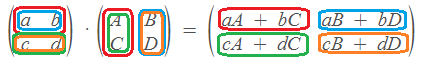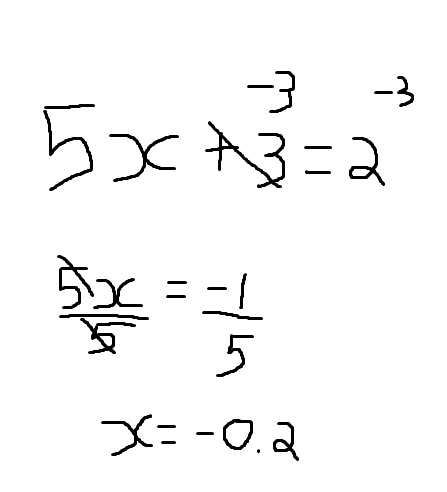# Help On CFrame:Inverse()

Hello,

I am trying to understand CFrame:Inverse()

What even is the inverse of a CFrame, and what is the formula used for getting the Inverse of a CFrame?

Thanks!Well CFrames are actually 4X4 matrices.

Inverse of a CFrame is another CFrame which when multiplied (matrix multiplication) with the original CFrame gives identity CFrame (ie CFrame.new(0, 0, 0, 1, 0, 0, 0, 1, 0, 0, 0, 1) or simply CFrame.new())

For instance:-
if ‘a’ is a CFrame, then
a * a:inverse() = CFrame.new()

If you want to know how to multiply matrices here is an example:-
(I used 2x2 matrix because I think it is the easiest to understand…)6 Likes

4 Likes

Please mark @ShakesRocker as the solution.But is there an actual formula for getting the inverse of a CFrame? For example if you have

``````local cf = CFrame.new(90.5, 0.5, 37.5, 0, 0, -1, 0, 1, 0, 1, 0, 0)

print(cf:Inverse())

- -> prints   -37.5, -0.5, 90.5, 0, 0, 1, 0, 1, 0, -1, 0, 0 how did it get these numbers?
``````

What does that word mean? [[30 characters]]

In context, I use it as a metaphor for how CFrames use the multiplication operation in practice. `CFrame1 * CFrame2:Inverse()` would feel very similar to doing `CFrame1 / CFrame2`, although that operation doesn’t quite exist.
It only carries weight in understanding how CFrames and their inverses interact with each other. No more, no less.

1 Like

Well finding the inverse of a CFrame is a lengthy and a messy process (well at least for me…)

But since you have asked… here you go:-

If you have read the CFrame math reference, you may have undertood how CFrame matrix works…

Here is a pic from the same reference page:-

now for understanding purpose say that in the above picture…
let cfa be a CFrame and let cfb be it’s inverse

So if you multiply them you get an identity CFrame…

So in the above pic if you compare the each component of the matrix in the LHS to it’s corresponding component of the identity CFrame matrix in the RHS, then you get a set of linear equations…

now since we know all the components in the original CFrame (in this case the original CFrame is cfa), as we have defined it…you can get all the components of it’s inverse CFrame as well (ie cfb in this case) by just solving these linear equations.

Now the point here is that, just so that programmers can avoid this lengthy procedure of finding the inverse of a CFrame… Roblox introduced CFrame:inverse()

Hopefully, that helped!3 Likes

What is LHS and RHS?
Edit: Oh, I’m assuming it means lefthandside/righthandside, don’t know how I didn’t notice that lol

LHS = Left hand side (expression at the left side of the equal-to symbol)
RHS = Right hand side (expression at the right side of the equal-to symbol)

Yes you are right about itI think I’m a little bit confused here… How do you get all the linear equations?
And also a linear equation is just an equation with a unknown value in it? For example a linear equation could be:and that would be how you solve it?

Yes, that is linear equation involving one variable…
In order to solve a linear equation with one variable you just need one equation

But for solving 2 variables you need 2 equation
for 3 you need 3, and so on…

Here is an simple example of how to solve a pair of linear equations (involving 2 variables)

Well in case of CFrame matrix you get a lot of linear equations…
(not sure but since there are 12 unknowns, there will be at least 12 linear equations, i think…)

1 Like

Can you please give me some different uses for CFrame:Inverse()? Thanks

Edit: Because I really don’t see the point in it …

1 Like

Well, I am really sorry but I personally have not used CFrame:inverse() much so I can’t be too sure about it.

Essentially it is used whenever we require to ‘divide’ 2 CFrames

cfa/cfb = cfa * cfb:inverse() (Since cfa/cfb is not possible)

where cfa and cfb are 2 CFrames.

I am not sure where we may encounter such a situation…

But you can go to the CFrame-Math-Operation (the link in my first reply) reference page where they gave an example of a rotating door where the offset of the door wrt the hinge is calculated using CFrame:inverse() so now the axis of rotation of the door is the vertical axis of the hinge

(But if it was me, I would have used a `Weld` or a `WeldConstraint` rather)